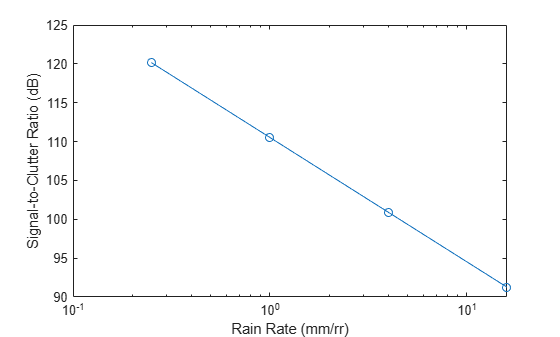# rainscr

Signal-to-clutter ratio due to rain

Since R2021a

## Syntax

``scr = rainscr(lambda,rrcs,tgtrcs,t)``
``scr = rainscr(lambda,rrcs,tgtrcs,t,vbrain)``

## Description

example

````scr = rainscr(lambda,rrcs,tgtrcs,t)` returns the signal-to-clutter ratio (SCR) due to rain.```

example

````scr = rainscr(lambda,rrcs,tgtrcs,t,vbrain)` specifies the rain velocity bandwidth.```

## Examples

collapse all

Estimate the signal-to-clutter-ratio due to rain of a side-looking airborne SAR. The SAR moves at 50 m/s in a direction orthogonal to the antenna boresight and operates at a frequency of 1.5 GHz. The rain rates are 0.25 mm/hr, 1 mm/hr, 4 mm/hr, and 16 mm/hr. The rain clutter volume is 20 m${}^{3}$. The SAR module has aperture processing length of 100 m. Assume the target RCS is 1 m${}^{2}$ and the velocity bandwidth of the rain is 4 m/s.

```v = 50; f = 1.5e9; lambda = freq2wavelen(f); rr = [0.25 1 4 16]; vol = 20; L = 100; tgtrcs = 1; vbrain = 4;```

Compute the signal-to-clutter ratio. Use `rainreflectivity` and `clutterVolumeRCS` to compute the rain radar cross-section. Use `sarinttime` to compute the aperture collection interval.

```volref = rainreflectivity(f,rr); rrcs = clutterVolumeRCS(volref,vol); t = sarinttime(v,L); scr = rainscr(lambda,rrcs,tgtrcs,t,vbrain);```

Plot the signal-to-clutter ratio as a function of the rain rate.

```semilogx(rr,scr,'o-') xlabel('Rain Rate (mm/rr)') ylabel('Signal-to-Clutter Ratio (dB)')```## Input Arguments

collapse all

Radar wavelength in meters, specified as a positive real scalar or a vector.

Data Types: `double`

Rain radar cross-section (RCS) in square meters, specified as a scalar or a vector.

Data Types: `double`

Target RCS in square meters, specified as a scalar.

Data Types: `double`

Aperture collection interval in seconds, specified as a positive real scalar.

Data Types: `double`

Rain velocity bandwidth in meters per second, specified as a positive scalar.

Data Types: `double`

## Output Arguments

collapse all

Signal-to-clutter ratio due to rain in decibels, returned as a matrix. The rows of `scr` correspond to the radar wavelength values in `lambda`. The columns of `scr` correspond to the rain RCS values in `rrcs`.

## Version History

Introduced in R2021a